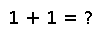trigamma

trigamma(x)

Compute the trigamma function of `x` (the logarithmic second derivative of `gamma(x)`)

Examples

Checking you are not a robot: